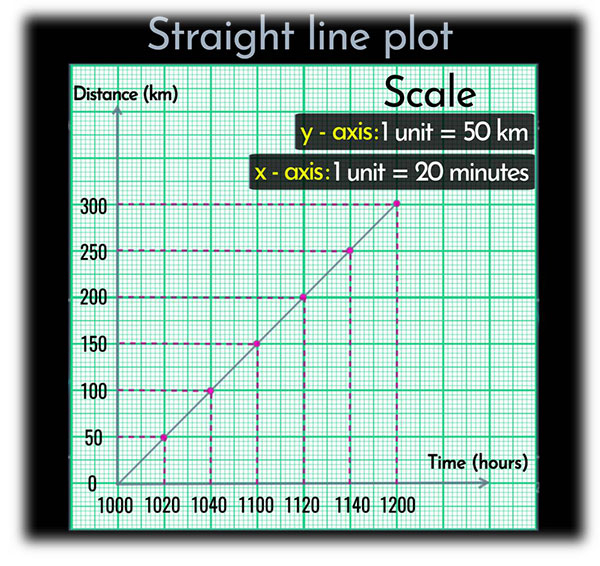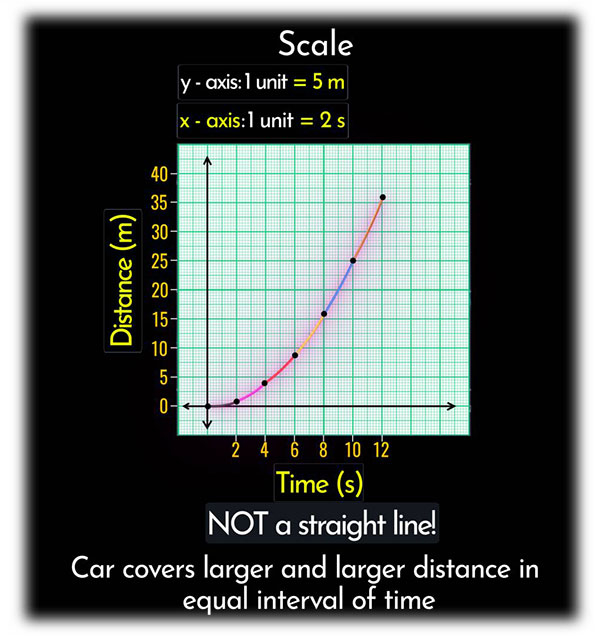# In the concept of distance ~ time graph,How we will draw a graph if the speed is not constant ?

Two cases are possible when speed is not constant.

Uniform Motion:

Uniform motion is a motion in which a body travels an equal distance in equal intervals of time. The nature of the distance-time graph for uniform motion in a straight line as shown below.Non-uniform motion:

But a non-uniform motion is a motion in which a body covers unequal distances in equal intervals of time. It is a curved path as shown below.Updated on: 10-Oct-2022

24 Views